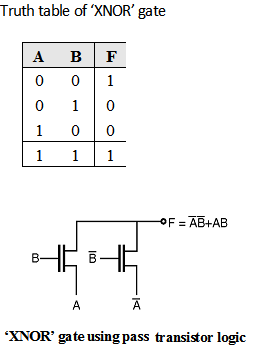Home > Digital CMOS Design > Pass Transistor Logic > XNOR gate using pass transistor logic

XNOR-gate-using-pass-transistor-logic | Pass-Transistor-Logic

'XNOR' gate using pass transistor logic. The truth table of 'XNOR' gate is as shown in Table below. In this gate if the B input is low then right NMOS transistor is ON and the inverted logic value of A is copied to the output F. When B input is high left NMOS transistor is ON and the logic value of A is copied to the output F, which satisfies the truth table of the XNOR gate.XNOR gate using transmission gate:

The Exclusive-OR (XOR) and equivalence functions can be implemented by using an input variable to control the transmission gates Recall that the exclusive-OR function is given by,

A  B = A  –B + –A  B Figure below shows the implementation of XNOR gate using transmission gate.